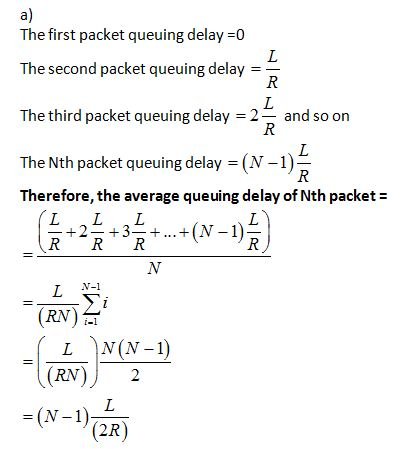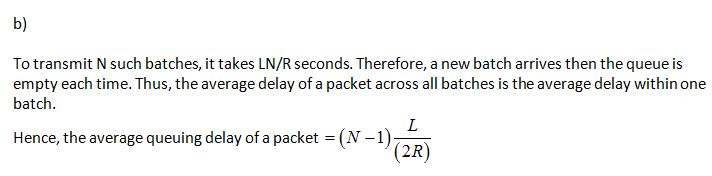SHARE
HELP

To start with, Sr2Jr’s first step is to reduce the expenses related to education. To achieve this goal Sr2Jr organized the textbook’s question and answers. Sr2Jr is community based and need your support to fill the question and answers. The question and answers posted will be available free of cost to all.Authors:
James F. Kurose, Keith W. Ross
Chapter:
Computer Networks And The Internet
Exercise:
Problems
Question:13 | ISBN:9780132856201 | Edition: 6

###Question

(a) Suppose N packets arrive simultaneously to a link at which no packets are currently being

transmitted or queued.Each packet is of length and the link has transmission rate R. What is

the average queuing delay for the N packets?

(b) Now suppose that N such packets arrive to the link every LN/R seconds.

What is the average queuing delay of a packet?

###Answer

Calculate the average queuing delay:###CroetCalc

a. What is the average queuing delay for the N packets? Should this question not be "What is the queuing delay of the Nth packet? If so then this is (N-1)*L/R

b. What is the average queuing delay of a packet? The average queuing delay for 1 packet when we have N packets will be (N-1)*(L/(2R))

Post the discussion to improve the above solution.